Data Preparation and Investigation

Preliminary Investigation

In your process flow diagram, select the Data Partition node. You can use the Variables property to access several summary statistics and statistical graphs for the input data set. Click the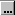button next to the Variable property of the Data Partition node. Select all 13 the variables and click Explore.
Tip
To select all of the variables, you can select one variable and press Ctrl+A on your keyboard.
The Explore window appears. This window contains the sample statistics for every variable, a histogram for each of the interval variables, and a bar chart for each class variable. This example highlights only a few of these plots, but you are encouraged to explore the rest on your own.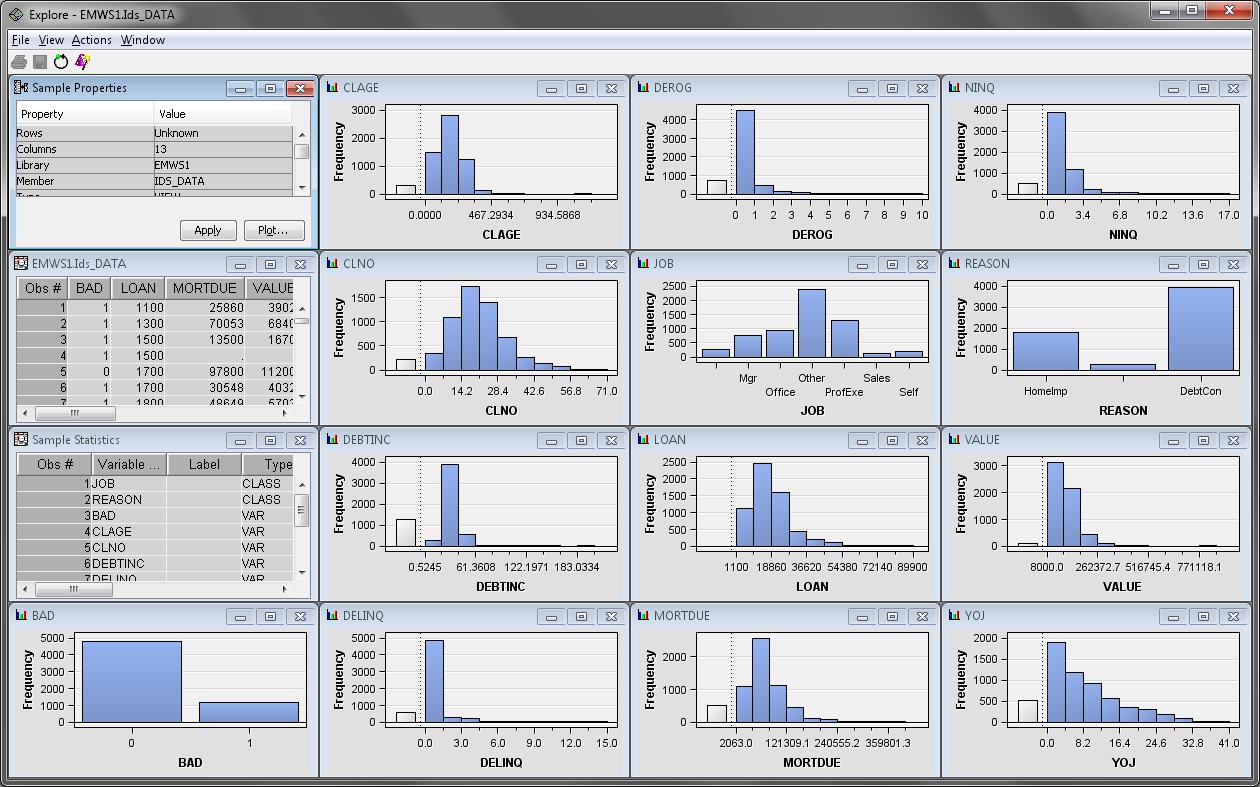Maximize the Sample Properties window. This window contains information about the data set sample that was used to create the statistics and graphics in the Explore window. The Fetched Rows property indicates the number of observations that were used in the sample. The SAMPSIO.HMEQ data set is small enough that the entire data set is used. Close the Sample Properties window.
Maximize the Sample Statistics window. This window contains the calculated mean, maximum, and minimum for the interval variables and the number of class levels, modal value, and percentage of observations in the modal value for class variables. The percentage of missing values is calculated for every variable. Close the Sample Statistics window.
Maximize the CLAGE window. The variable CLAGE indicates the age of the client’s oldest credit line in months.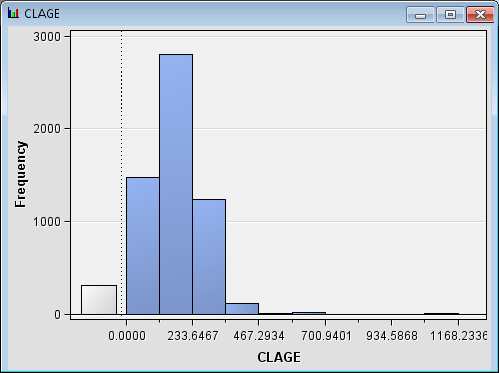The gray bar on the left side of the histogram represents the missing values. Notice that the vast majority of the observations are less than 350. The CLAGE data set is skewed right. Close the CLAGE window.
Maximize the REASON window. This variable represents the stated reason why the client took out the loan.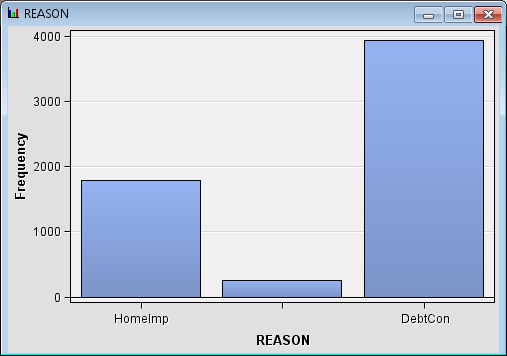The unlabeled bar in this bar chart indicates the missing values. Notice that significantly more people listed debt consolidation as the reason that they borrowed instead of home improvement. Close the REASON window.
It is highly encouraged that you view the distributions for each of the remaining variables. When you are finished, close the Explore window. Next, close the Variables window.

Performing Variable Transformation

After you have viewed the sample statistics and variable distributions, it is obvious that some variables have highly skewed distributions. In highly skewed distributions, a small percentage of the data points can have a large amount of influence on the final model. Sometimes, performing a transformation on an input variable can yield a better fitting model. The Transform Variables node enables you to perform variable transformation.
From the Modify tab, drag a Transform Variables node to your diagram workspace. Connect the Data Partition node to the Transform Variables node. Clicknext to the Variables property of the Transform Variables node. The Variables window appears.
In the Variables window, select the Statistics option in the upper right corner of the screen. Scroll the variables list all the way to the right. You should see the Skewness and Kurtosis statistics.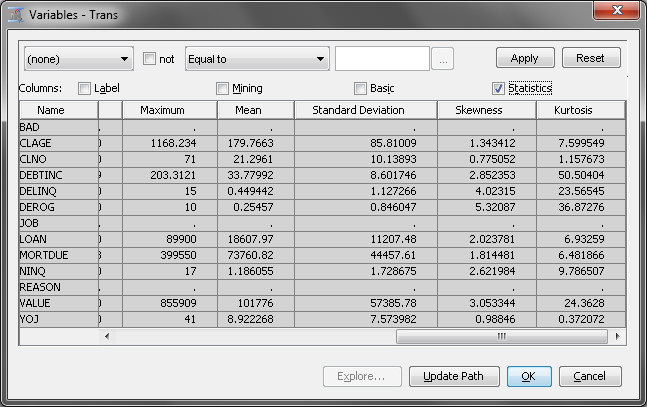The Skewness statistic indicates the level of skewness and the direction of skewness for a distribution. A Skewness value of 0 indicates that the distribution is perfectly symmetrical. A positive Skewness value indicates that the distribution is skewed to the right, which describes all of the variables in this data set. A negative value indicates that the distribution is skewed to the left.
The Kurtosis statistic indicates the peakedness of a distribution. However, this example focuses only on the Skewness statistic.
The Transform Variables node enables you to rapidly transform interval variables using standard transformations. You can also create new variables whose values are calculated from existing variables in the data set. Note that the most skewed variables are, in order, DEROG, DELINQ, VALUE, DEBTINC, NINQ, and LOAN. These five variables all have a Skewness value greater than 2. Close the Variables window.
This example applies a log transformation to all of the input variables. The log transformation creates a new variable by taking the natural log of each original input variable. In your diagram workspace, select the Transform Variables node. Set the value of the Interval Inputs property to Log.
Right-click the Transform Variables property and click Run. Click Yes in the Confirmation window. In the Run Status window, click Results.
Maximize the Transformations Statistics window. This window provides statistics for the original and transformed variables. The Formula column indicates the expression used to transform each variable. Notice that the absolute value of the Skewness statistic for the transformed values is typically smaller than that of the original variables. Close the Results window.
The Transform Variables enables you to perform a different transformation on each variable. This is useful when your input data contains variables that are skewed in different ways. In your diagram workspace, select the Transform Variables node. Clicknext to the Variables property of the Transform Variables node. The Variables window appears. In the Variables window, note the Method column. Use this column to set the transformation for each variable individually.
Before doing so, you want to recall the distribution for each variable. Select the variable DEROG and click Explore. Note that nearly all of the observations have a value of 0. Close the Explore window.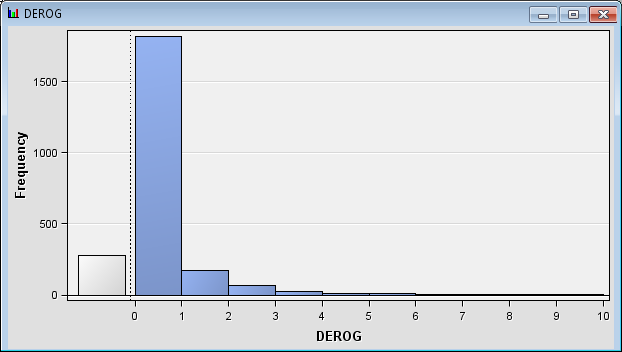Repeat this process for the DELINQ variable. Nearly all of the values for DELINQ are equal to 0. The next largest class is the missing values.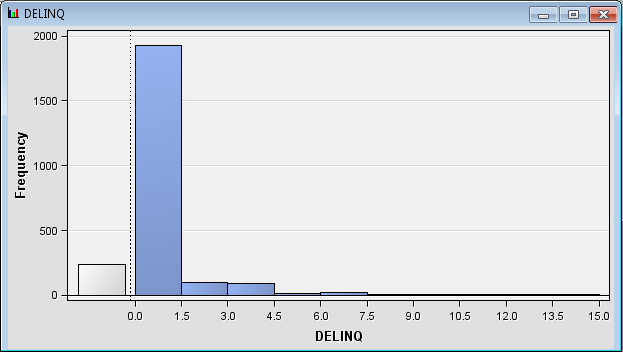In situations where there is a large number of observations at one value and relatively few observations spread out over the rest of the distribution, it can be useful to group the levels of an interval variable. Close the Variables window.
Instead of fitting a slope to the whole range of values for DEROG and DELINQ, you need to estimate the mean in each group. Because most of the applicants in the data set had no delinquent credit lines, there is a high concentration of observations where DELINQ=0.
In your process flow diagram, select the Transform Variables node. Clicknext to the Formulas property. The Formulas window appears.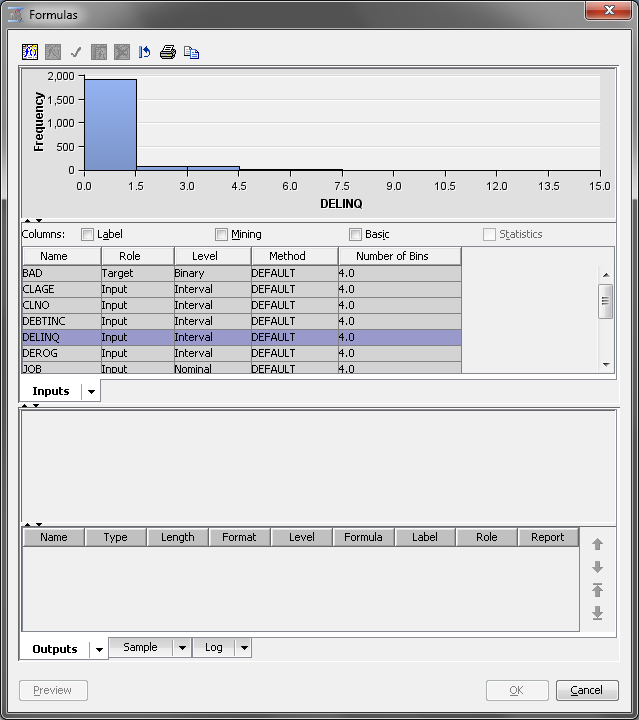The Formulas window enables you to create custom variable transformations. Select the DELINQ variable and click the Create variable in the upper left corner of the Formulas window. The Add Transformation window appears.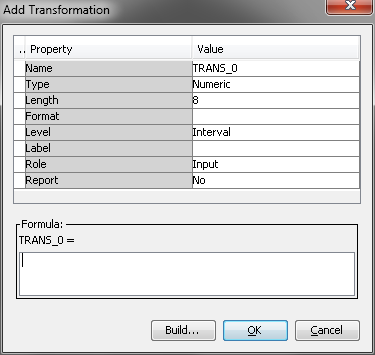Complete the following steps to transform the DELINQ variable:
1. Enter `INDELINQ` for the Name property. The default values are acceptable for the other properties.
2. In the Formula dialog box, enter `DELINQ > 0`.
This definition is an example of Boolean logic and illustrates one way to dichotomize an interval variable. The statement is either true or false for each observation. When the statement is true, the expression evaluates as 1. Otherwise, the expression evaluates as 0. In other words, when DELINQ>0, INDELINQ=1. Likewise, when DELINQ=0, INDELINQ=0. If the value of DELINQ is missing, the expression evaluates to 0, because missing values are treated as being smaller than any nonmissing values in a numerical comparison. Because a missing value of DELINQ is reasonably imputed as DELINQ=0, this does not pose a problem for this example.
3. Click OK. The formula now appears in the Formulas window.
4. Repeat the above steps for the variable DEROG. Name the new variable `INDEROG`.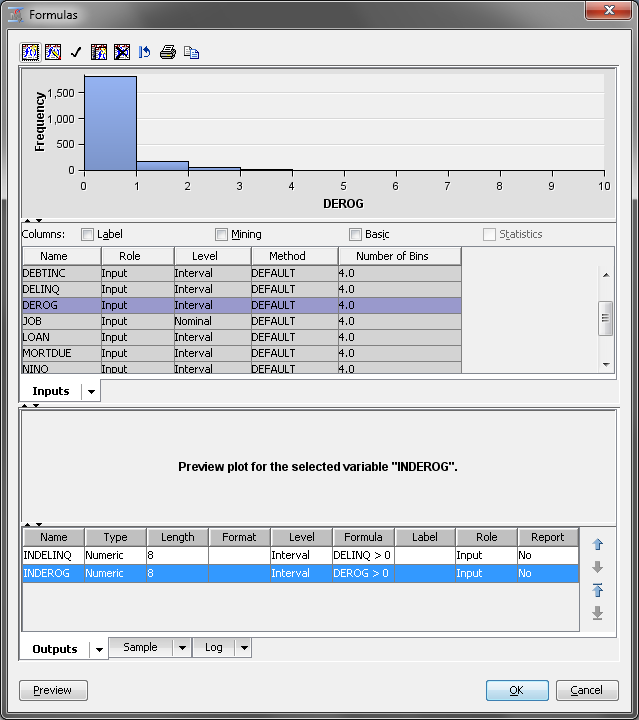5. Click Preview in the lower left corner of the screen.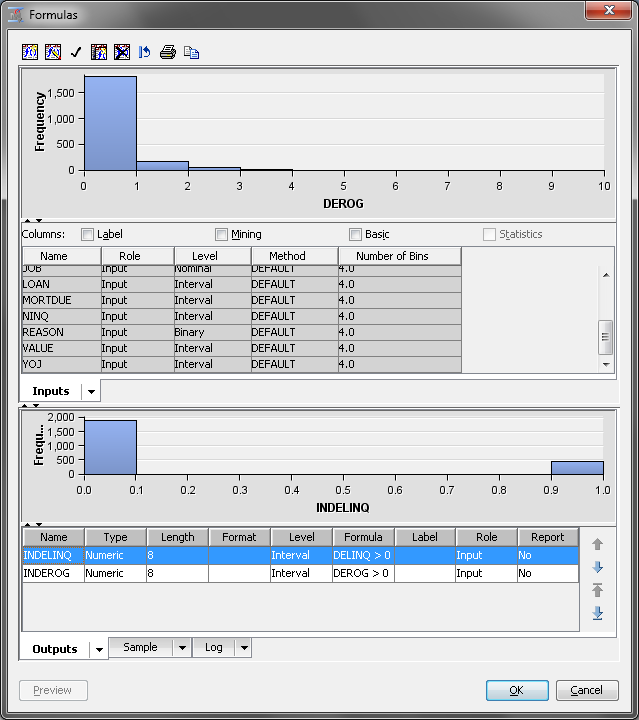6. Click OK.
Even though DEROG and DELINQ were used to construct the new variables, the original variables are still available for analysis. You can modify this if you want, but this example keeps the original variables. This is done because the transformed variables contain only a portion of the information that is contained in the original variables. Specifically, the new variables identify whether DEROG or DELINQ is greater than zero.

Understanding Interactive Binning

An additional processing technique to apply before modeling is binning, also referred to as grouping. The Interactive Binning node enables you to automatically group variable values into classes based on the node settings. You have the option to modify the initially generated classes interactively. By using the Interactive Grouping node, you can manage the number of groups of a variable, improve the predictive power of a variable, select predictive variables, generate the Weight of Evidence (WOE) for each group of a variable, and make the WOE vary smoothly (or linearly) across the groups.
The WOE for a group is defined as the logarithm of the ratio of the proportion of nonevent observations in the group over the proportion of event observations in the group. For the binary target variable BAD in this example, BAD=1 (a client who defaulted) is the event level and BAD=0 (a client who repaid the loan) is the nonevent level. WOE measures the relative risk of a group. Therefore, high negative values of WOE correspond to high risk of loan default. High positive values correspond to low risk.
After the binning of variable values has been defined for a variable, you can assess the predictive power of the variable. The predictive power of a variable is the ability of the variable to distinguish event and nonevent observations. In this example, it is the ability to separate bad loan clients from good loan clients. You can assess the predictive power by using one of the following criteria:
• Information Value — is the weighted sum of WOE over the groups. The weight is the difference between the proportion of nonevents and the proportion of events in each group.
• Gini Score — is the same as the Gini index in the Tree node. See the Interactive Grouping node in the SAS Enterprise Miner Help for more information.
The WOE variables are usually used as inputs in successor modeling nodes.

Performing Interactive Binning

Recall the distribution of NINQ. NINQ is a counting variable, but the majority of the observations have the value of either 0, 1, or 2. It might be useful to create a grouped version of NINQ by pooling all of the values larger than 2 (of which there are very few) into a new level. This would create a new three-level grouping variable from NINQ. Creating a grouping variable that has three levels causes loss of information about the exact number of recent credit inquiries. But it does enable you to handle nonlinearity in the relationship between NINQ and the response variable.
In order to bin NINQ, you need to add an Interactive Binning node to your process flow diagram. However, because you plan to bin NINQ, you do not want to transform it with the Transform Variables node. Right-click the Transform Variables node and click Variables. Set the value of Method for the variable NINQ to None. The Transform Variables node will not transform NINQ.
On the Modify tab, drag an Interactive Binning node to your process flow diagram. Connect the Transform Variables node to Interactive Binning node.
Right-click the Interactive Binning node and click Run. In the Confirmation window, click Yes. In the Run Status window, click OK.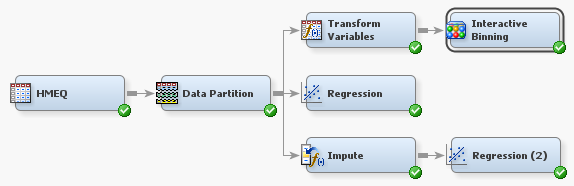In your diagram workspace, select the Interactive Binning node. Next, clicknext to the Interactive Binning property in the properties panel.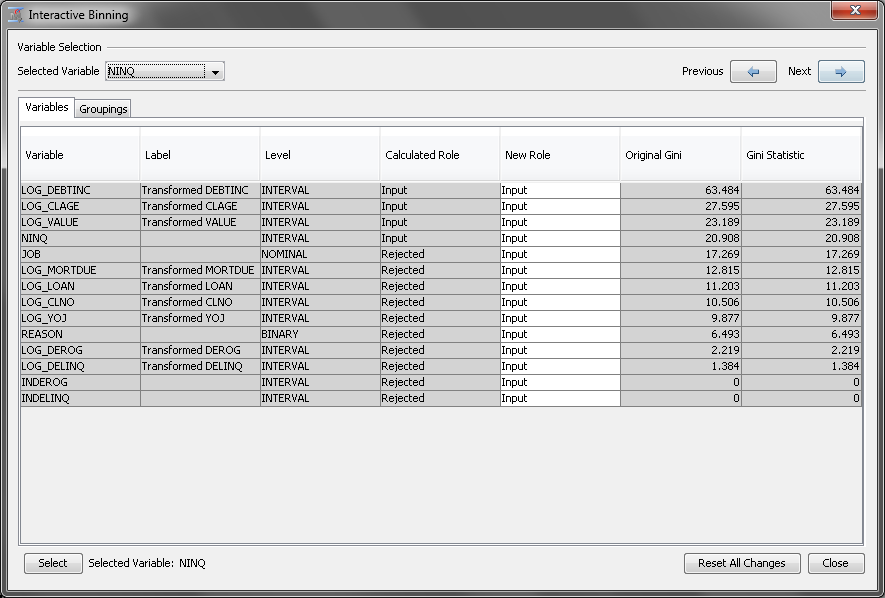Use the Interactive Binning window to manually bin the input variables. Before binning NINQ, first notice that most of the variables have a Calculated Role of Rejected. Because you want to use these variables in the data mining process, set their New Role to Input. To do so, select all of the variables. Then right-click anywhere in the selection and click Input. This result is shown in the image above.
Next, on the Selected Variable drop-down menu, located in the upper left corner of the screen, click NINQ. Next, click the Groupings tab to see details about the variable NINQ.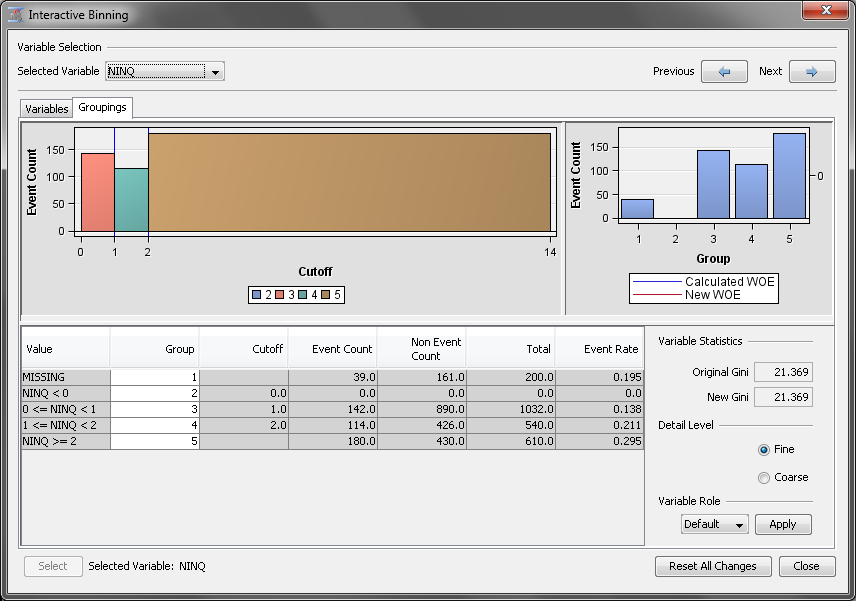Automatically, the Interactive Binning node created five groups for NINQ. Notice, however, that the first two groups contain the missing values and negative values, respectively. Also notice that the group that contains the negative values is empty. For this example, you assume that a missing value indicates that no credit inquiry was made. Therefore, you want to merge both of these groups into the third group.
First, select the MISSING group and right-click that row. Click Assign To. In the Group Selection window, select 3 for the Select a Group selection.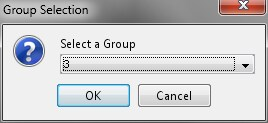Notice that the groups were renumbered.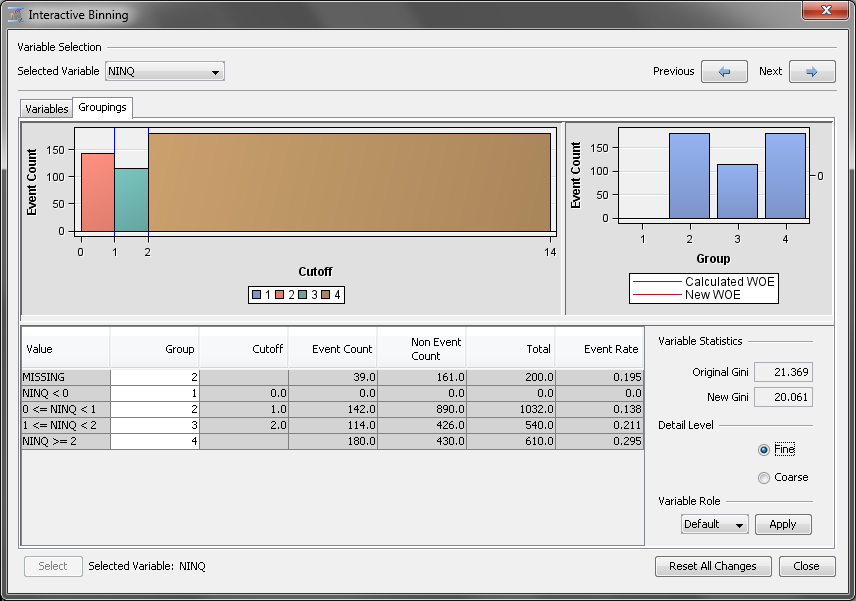Next, repeat this process for the empty bin that contains the negative values. Select the group NINQ < 0 and right-click that row. Click Group = 2. Once again, this renumbers the groups.
You now have three groups for the variable NINQ. But you want to add a fourth group that contains all of the values greater than two and retain a group that contains just 2. To do so, select the row NINQ >= 2 and right-click that row. Click Split Bin. Enter `2.5` in the Enter New Cutoff Value dialog box of Split Bin window. This creates a separate bin that still belongs to the same group. To assign this new bin to its own group, right-click the row 2.5 <= NINQ and click Group = 4.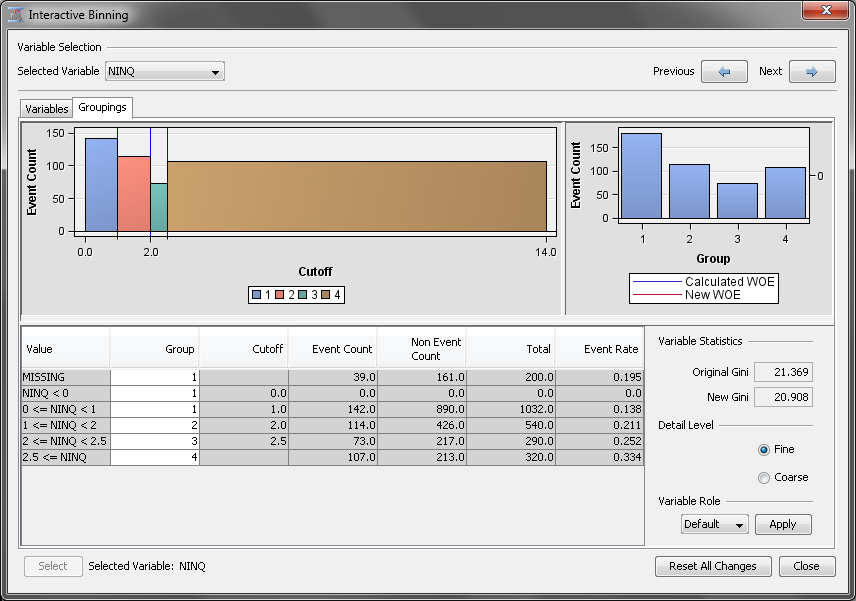Note: Because the values of NINQ are only whole numbers, you could have selected any value between 2 and 3, exclusive as the new cutoff value.
On the Select Variable drop-down menu, click INDEROG. Notice that the Interactive Grouping node decided to split INDEROG at 0, which means that every observation now belongs to the same bin. Select the row INDEROG >= 0, right-click that row and click Split Bin. Enter `0.5` in the Enter New Cutoff Value dialog box. Click OK. Now right-click the row 0.5 <= INDEROG and click GROUP = 4.
You need to repeat this same process for the variable INDELINQ. On the Select Variable drop-down menu, click INDELINQ. Right-click the row INDELINQ >= 0, and click Split Bin. Enter `0.5` in the Enter New Cutoff Value dialog box. Click OK. Now right-click the row 0.5 <= INDELINQ and click GROUP = 4.
For both INDELINQ and INDEROG, group 3 corresponds to an original value of 0 and group 4 corresponds to an original value of 1.
Click Close to close the Interactive Binning window. Click Yes in the Save Changes window.